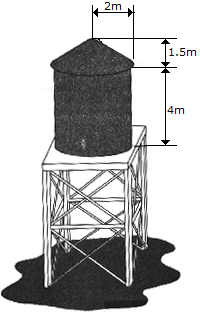# Engineering Mechanics - Center of Gravity and Centroid - Discussion

### Discussion :: Center of Gravity and Centroid - General Questions (Q.No.1)

1.Determine the approximate amount of paint needed to cover the surface of the water storage tank. Assume that a liter of paint covers 2.5 m2. Also, what is the total inside volume of the tank.

 [A]. 27.6 liters of paint, V = 52.6 m3 [B]. 20.1 liters of paint, V = 50.3 m3 [C]. 26.4 liters of paint, V = 56.5 m3 [D]. 25.1 liters of paint, V = 55.0 m3

Explanation:

No answer description available for this question.

 Arpit Lasod said: (Jan 5, 2011) volume of cylinder = pi*r*r*h = pi*4*4 = 50.26 volume of cone = 1/3*pi*r*r*h = 0.333*pi*4*1.5 = 6.27 volume of cylinder + volume of cone = 56.53

 B Mccormick said: (Jan 19, 2012) Surface area of cylinder = pi*d*height 3.14x4x4=50.2m2 Surface area of cone = pi*r*s s=hyp of triangle s=sqr root(2^2+1.5^2) s= 2.5m pi*r*s = 15.7m2 total = 50.2+15.7=65.9m2 /2.5m2 = 26.4 liters of paint

 Rajkaran Vaishya said: (Oct 1, 2012) Engineering mechanics is good for us. That problem is very hard.

 Shiv Bhardwaj said: (Jun 4, 2013) Volume of cylinder volume of cone = Total volume. & you know very well :).

 Aniket said: (Aug 17, 2014) Amount of paint required is total surface of tank divided by the area paint covers in 1 l; worked out that comes to 26.4 l, had to refer to a site for knowing surface area of cone ;); Also, for painting do we need to consider base surface of tank?

 Rohit Nandan Tiwari said: (Sep 3, 2015) Please anyone tell me about how much liters of paint in use.

 Mithun Kumar said: (Dec 2, 2015) Volume = Volume of cylinder+Volume of cone = 56.53 m^3.

 Mohana Vignesh said: (Nov 22, 2016) The volume of cylinder = π * r * r * h = π * 4 * 4 = 50.26. The volume of cone = 1/3* π * r * r * h = 0.333 * π * 4 * 1.5 = 6.27. The volume of cylinder + volume of cone = 56.53.